# Area of Triangles Lesson Plan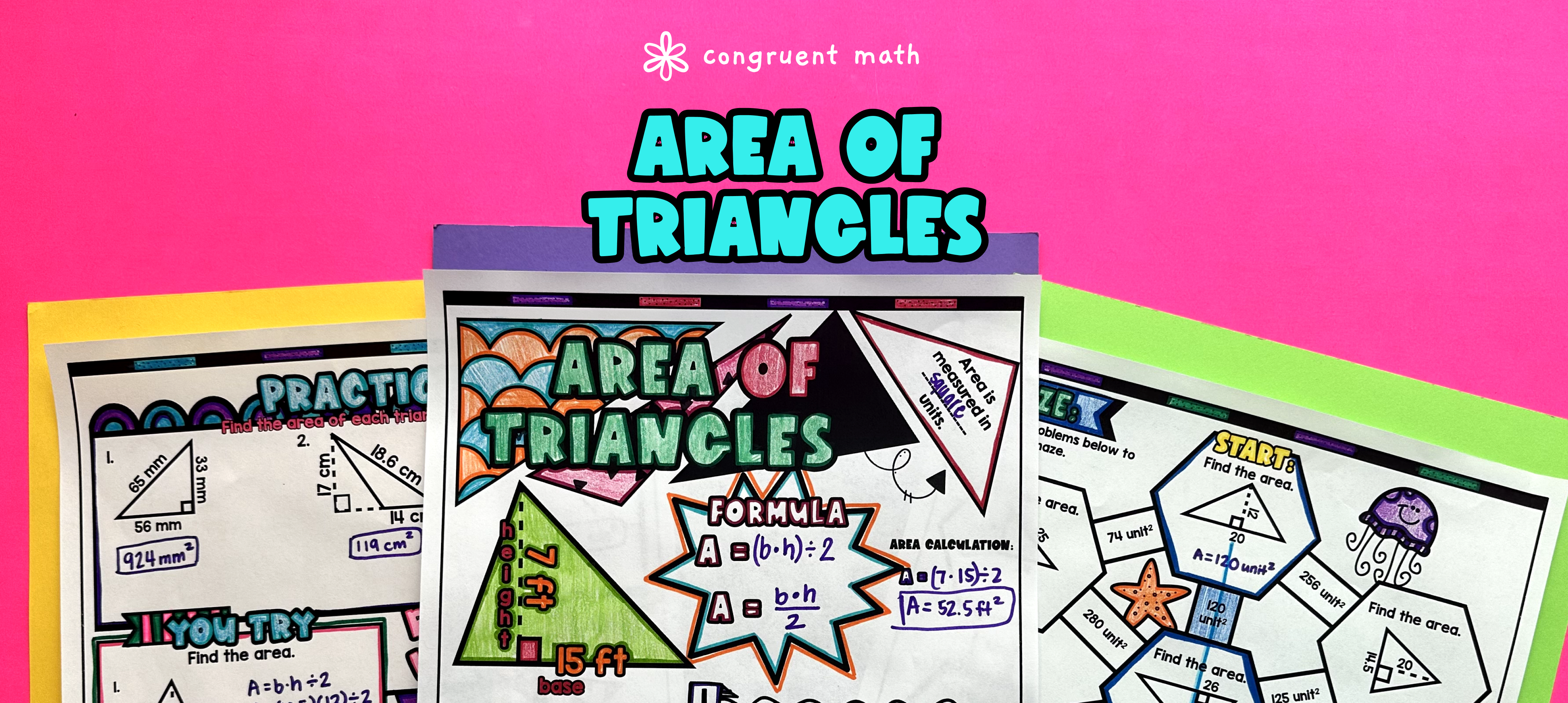Pin This

## Overview

Ever wondered how to teach the area of triangles in an engaging way to your sixth and seventh grade students?

In this lesson plan, students will learn about calculating the area of triangles and their real-life applications. Through artistic, interactive guided notes, check for understanding, a doodle & color by number activity, and a maze worksheet, students will gain a comprehensive understanding of finding the area of triangles.

The guided notes will provide structured instructions on how to calculate the area of triangles while integrating checks for understanding to ensure students are on the right track.

The lesson culminates with a real-life example that explores the application of finding the area of triangles in everyday situations. This will help students see the relevance and importance of the topic in real-world scenarios.

## Get the Lesson Materials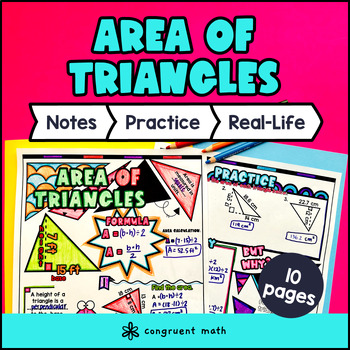\$4.25

## Learning Objectives

After this lesson, students will be able to:

• Calculate the area of a triangle using the formula A = 1/2 * base * height

• Recognize and describe real-life applications of the area of triangles, such as in construction or design

## Prerequisites

Before this lesson, students should be familiar with:

• Knowledge of basic mathematical operations (addition, subtraction, multiplication, and division) for whole numbers and decimals

## Key Vocabulary

• Area of triangles

• Base

• Height

## Procedure

### Introduction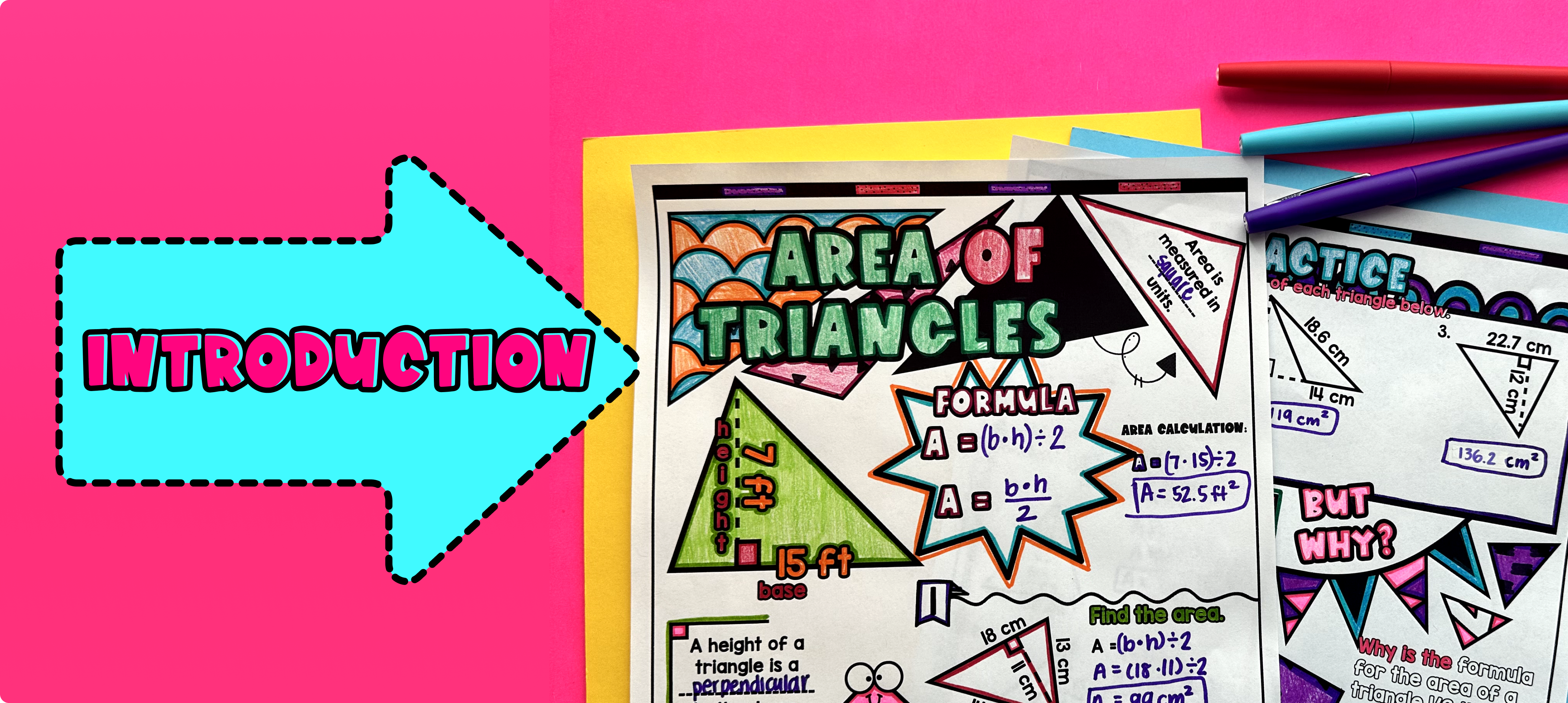Pin This

As a hook, ask students why is it important to calculate the area of triangles in real-life situations. Use the first page of the guided notes to introduce the topic of calculating the area of triangles. Walk through the key points of the topic of the guided notes, which includes:

• Introducing the formula for calculating the area of a triangle

• Explaining how to identify the base and height of a triangle

• Discussing the relationship between the area of a triangle and a rectangle

Based on student responses, reteach concepts that students need extra help with. If your class has a wide range of proficiency levels, you can pull out students for reteaching and have more advanced students begin work on the practice exercises.

### Practice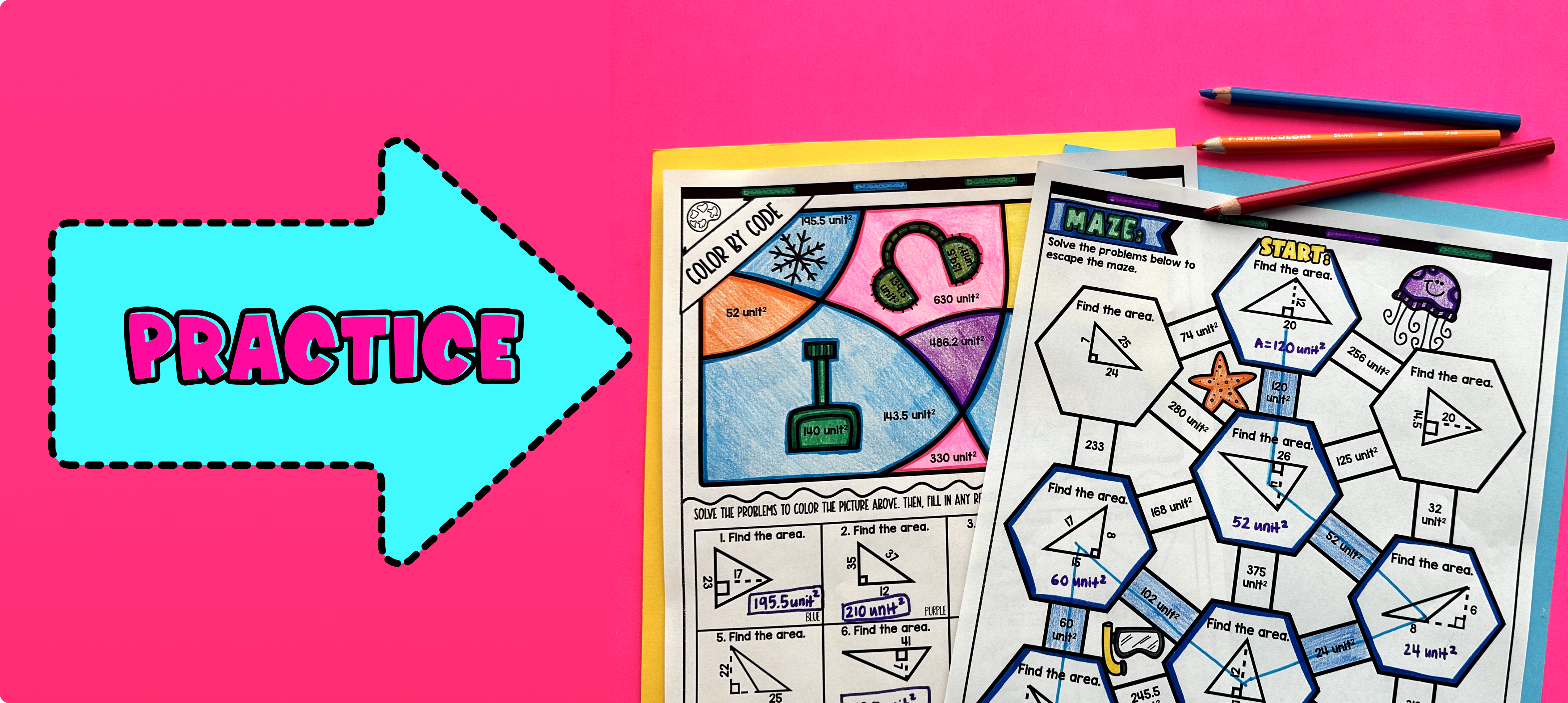Pin This

Have students practice calculating the area of triangles using the practice sheet (page 2 of guided notes) and Color by Code worksheet (page 3 of guided notes)

Walk around the classroom to answer any questions students may have and provide support as needed.

For students who finish the Color by Code worksheet quickly, they can work on the maze activity (page 4 of guided notes) for additional practice. You can also assign the maze activity as homework for students to complete for the remainder of the class.

### Real-Life Application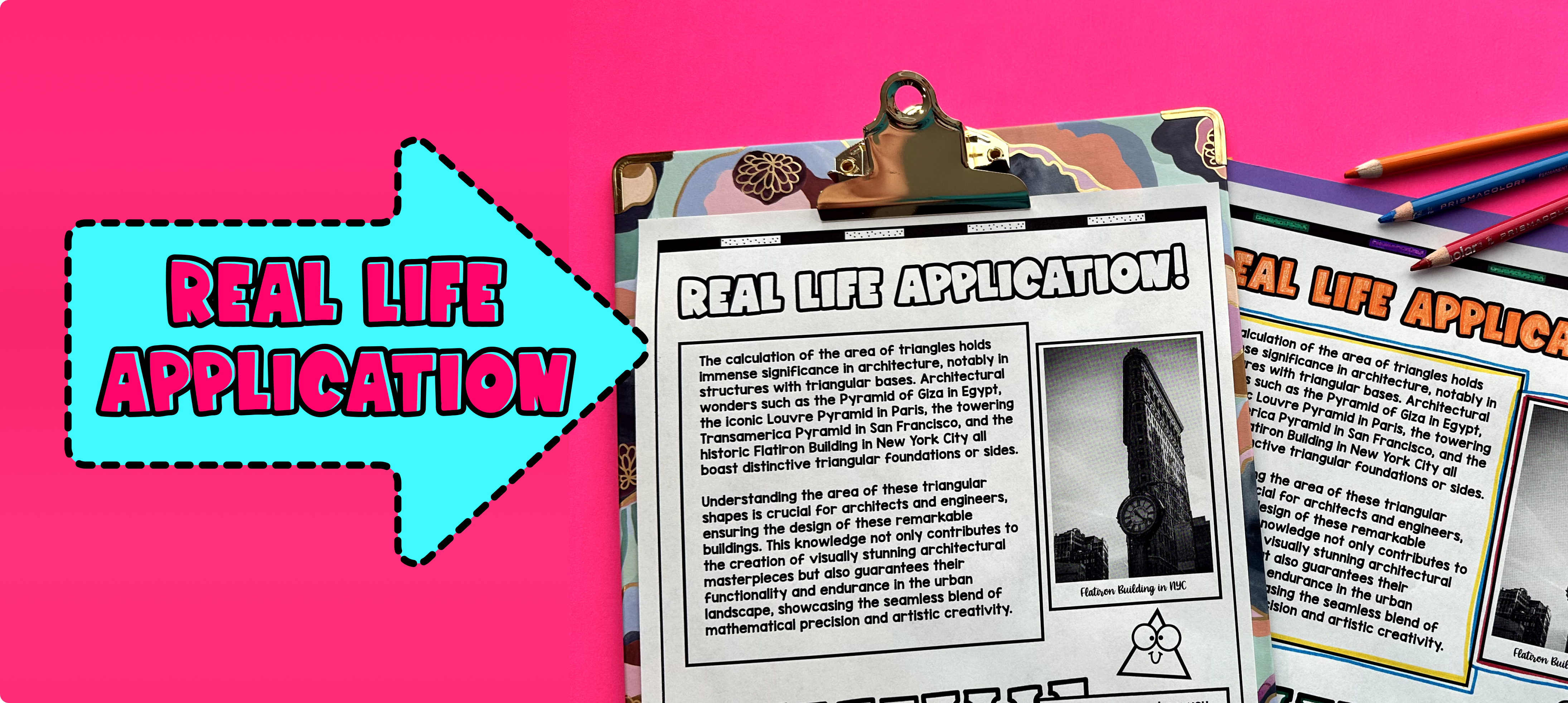Pin This

Use the last page of the guided notes "real life applications" to bring the class back together, and introduce the concept of real-world applications of the area of triangles. Explain to the students that the area of triangles is not just a mathematical concept, but it also has practical applications in our everyday lives.

Some examples of real-life applications of the area of triangles include:

1. Construction: Architects and engineers often use the area of triangles in their designs. For example, when designing a roof, they need to calculate the area of triangular sections to determine the amount of material needed.

2. Landscaping: Landscapers need to calculate the area of triangular flowerbeds or patches of grass to determine the amount of soil or sod required.

3. Architecture: Ancient civilizations, such as the Egyptians and the Greeks, used the area of triangles in the construction of their iconic buildings and structures. The pyramids in Egypt, for instance, are built with triangular faces.

These are just a few examples of how the area of triangles is used in the real world. It is important for students to understand the practical significance of this mathematical concept and how it applies to various fields and disciplines.

## Extensions

If you’re looking for digital practice for Area of Triangles, try my Pixel Art activities in Google Sheets. Every answer is automatically checked, and correct answers unlock parts of a mystery picture. It’s incredibly fun, and a powerful tool for differentiation.

Here’s a few activities to explore: